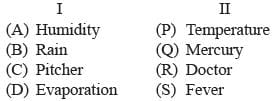Test: Analogy- 1

# Test: Analogy- 1 - Class 10

Test Description

## 10 Questions MCQ Test Olympiad Preparation for Class 10 - Test: Analogy- 1

Test: Analogy- 1 for Class 10 2023 is part of Olympiad Preparation for Class 10 preparation. The Test: Analogy- 1 questions and answers have been prepared according to the Class 10 exam syllabus.The Test: Analogy- 1 MCQs are made for Class 10 2023 Exam. Find important definitions, questions, notes, meanings, examples, exercises, MCQs and online tests for Test: Analogy- 1 below.
Solutions of Test: Analogy- 1 questions in English are available as part of our Olympiad Preparation for Class 10 for Class 10 & Test: Analogy- 1 solutions in Hindi for Olympiad Preparation for Class 10 course. Download more important topics, notes, lectures and mock test series for Class 10 Exam by signing up for free. Attempt Test: Analogy- 1 | 10 questions in 20 minutes | Mock test for Class 10 preparation | Free important questions MCQ to study Olympiad Preparation for Class 10 for Class 10 Exam | Download free PDF with solutions
 1 Crore+ students have signed up on EduRev. Have you?
Test: Analogy- 1 - Question 1

### In the following questions, choose the word that shows the same relationship as given in the each question. Lira is related to Italy in the same way as Taka is related to

Test: Analogy- 1 - Question 2

### In the following questions, choose the word that shows the same relationship as given in the each question. Disease is  related to pathology in the same way as Planet is related to

Test: Analogy- 1 - Question 3

### In the following questions, choose the word that shows the same relationship as given in the each question. Match is  related to Win in the same way as Examination is related to

Test: Analogy- 1 - Question 4

In each of the following questions, there are two terms to the left of the sign :: which are related in some way. Obtain the same relationship between the term to the right of the sign :: from one of the four alternatives given under it.
? : CEIG :: LNRP : OKUM

Test: Analogy- 1 - Question 5

In each of the following questions, there are two terms to the left of the sign :: which are related in some way. Obtain the same relationship between the term to the right of the sign :: from one of the four alternatives given under it.
LJPN : KMOQ :: ? : XVTZ

Test: Analogy- 1 - Question 6

In each of the following questions, there are two terms to the left of the sign :: which are related in some way. Obtain the same relationship between the term to the right of the sign :: from one of the four alternatives given under it.​
AZB : BYC :: CXD : ?

Test: Analogy- 1 - Question 7

In each of the following questions, there are two terms to the left of the sign :: which are related in some way. Obtain the same relationship between the term to the right of the sign :: from one of the four alternatives given under it.
JTIS : HRGQ :: FPEO : ?

Test: Analogy- 1 - Question 8

In each of the following questions, two words indicated by I and II have been left out.The correct word to come in place of I is given as one of the four alternatives against I and the correct words to come in place of II are given as one of the four alternatives against II. Read the correct words, there is some relationship between the two words to the left of the sign (: :) and the same relationship exist between the two words to the right of the sign (: :). The correct combination is given as one of the four alternatives (A), (B), (C) and (D). Find the correct combination in each case.
I : Water : : Thermometer : IIDetailed Solution for Test: Analogy- 1 - Question 8

First contains the second.

Test: Analogy- 1 - Question 9

Select the pair in which the numbers are similarly related as in the given pair. 11 : 1210

Test: Analogy- 1 - Question 10

In the question, choose the set of numbers from the alternative set that is similar to the given set.
Given set: (246, 257, 358)

Detailed Solution for Test: Analogy- 1 - Question 10

Correct option is C.
The sum of digits of the numbers in a set are 12, 14 and 16 respectively.

## Olympiad Preparation for Class 10

11 videos|36 docs|201 tests
Information about Test: Analogy- 1 Page
In this test you can find the Exam questions for Test: Analogy- 1 solved & explained in the simplest way possible. Besides giving Questions and answers for Test: Analogy- 1, EduRev gives you an ample number of Online tests for practice

## Olympiad Preparation for Class 10

11 videos|36 docs|201 tests